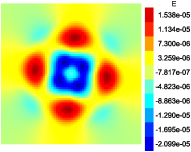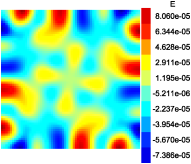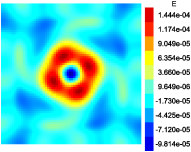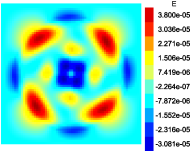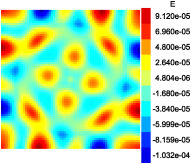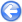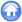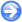Author
Stéphane Popinet
Command
sh nonlinear.sh
Version
120814
Required files
nonlinear.sh river.gfs ocean.gfs error.gfv error-ocean.gfv error-1.ref error-ocean-1.ref error-river-1.ref
Running time
7 minutes 50 seconds

For a circular vortex defined by a tangential velocity uθ(r), the radial height/pressure profile is a solution of

h0(r)=
 uθ g

2Ω +
 uθ r

with Ω the angular velocity. For this test case we take

 uθ(r)=(r < 0.4)є(1+cos(π(r−0.2)/0.2))/2

The control parameters are the Froude number U/√gH and the Rossby number Ro=UL. We set the Froude number to 0.1 and consider Rossby numbers 0.1 and ∞ (no rotation). In the case without rotation the errors reflect only the accuracy of the momentum advection terms. With rotation, the errors also depend on the accuracy of the discretisation of the geostrophic balance.

Figure 133 illustrate the evolution of the errors on free surface height/pressure for the different solvers, with and without rotation.

 Figure 133: Evolution of the maximum relative error on free-surface height. (a) Ro=∞, (b) Ro=0.1.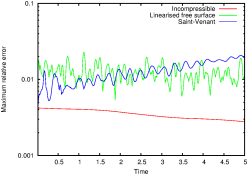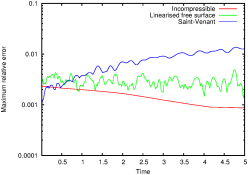(a) (b)

Figure 134 display the error distributions at t=5.

 Figure 134: Error distribution at t=5. Top row: Ro=∞, bottom row: Ro=0.1.
 incompressible linearised free-surface Saint-Venant